### Total 39 Videos found in Category "Pre-algebra"

Title
 1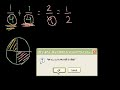Adding and subtracting fractions 2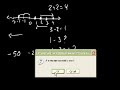Adding/Subtracting negative numbers 3Addition and Subtraction with Significant Figures 4Binary Numbers 5Binary, Decimal and Hexadecimal Number Systems 6Common Divisibility Examples 7Comparing Absolute Values 8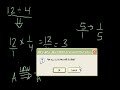Dividing fractions 9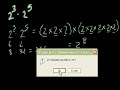Exponent Rules Part 1 10Exponent Rules Part 2 11Interpreting Linear Graphs 12Introduction to logarithm properties 13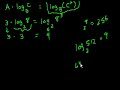Introduction to logarithm properties (part 2) 14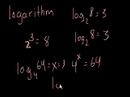Introduction to Logarithms 15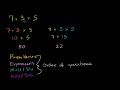Introduction to Order of Operations 16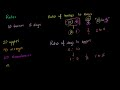Introduction to Ratios (new HD version) 17Inverse Property of Addition 18Inverse Property of Multiplication 19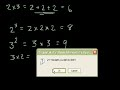Level 1 Exponents 20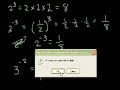Level 2 Exponents 21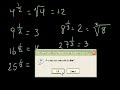Level 3 exponents 22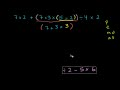More Complicated Order of Operations Example 23More on Significant Figures 24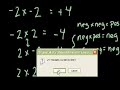Multiplying and dividing negative numbers 25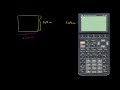Multiplying and Dividing with Significant Figures 26Multiplying fractions (old) 27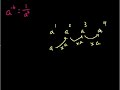Negative Exponent Intuition 28Negative Numbers Introduction 29Number Base Conversion Practice 30Ordering Fractions 31Ordering Negative Numbers 32Pure Numbers and Significant Digits 33Scientific Notation (old) 34Scientific Notation Examples 35Significant Figures 36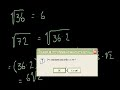Simplifying radicals 37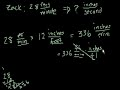Speed translation 38Unit conversion 39Unit Conversion Example: Drug Dosage

Say and share some thing about these videos...